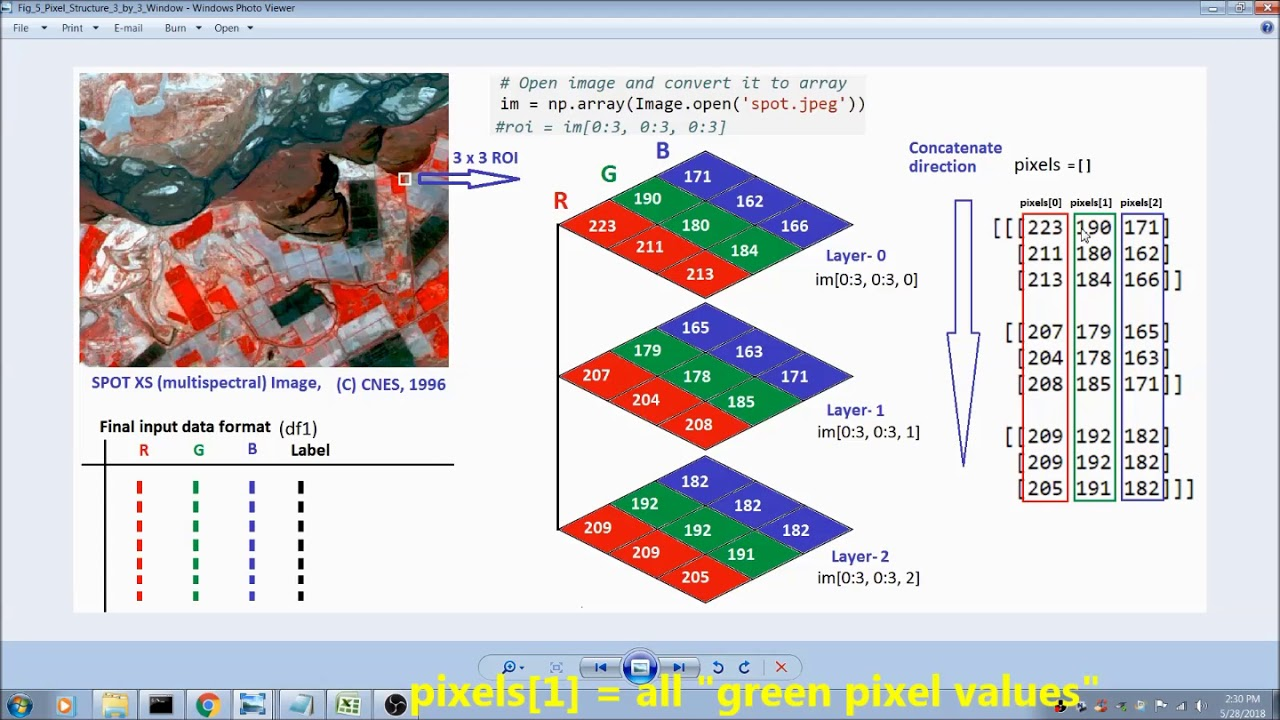# Image Classifier Using Machine Learning, Neural Networks-For Medical/Satellite Images #1In this tutorial, image classification, classify a single image into several categories, by using machine learning/neural networks has been presented. There are many machine learning algorithms which can be applied to image recognition or classifications.

There are many machine learning algorithms which can be applied to image recognition or classifications. In most of them, an image will be input to the network, then the input image will be classified into one of the predefined output categories. In this tutorial, image classification, classify a single image into several categories, by using machine learning/neural networks has been presented. The algorithm can be applied to other image types including medical images (if we use medical images to train the same neural network).

## Different Optimization Algorithm for Deep Neural Networks: Complete Guide

In this article, we will only focus on the Better Optimizing algorithm for Deep Neural Network (DNN). We will call this optimizing algorithm as a Learning algorithm for this article.

## How to Learn Any Algorithm in Machine Learning

If you are new to the field, the best way to develop a firm understanding of the fundamentals is quite simple. Simply put, while you are learning the algorithm, attempt to build it from scratch in your favorite programming language. Allow me to explain…

## What Is Model & Algorithm In Machine Learning | Machine Learning Tutorials | Python 04

What Is Model & Algorithm In Machine Learning | Machine Learning Tutorials | Python | Ml Python

## Reptile: OpenAI’s Latest Meta-Learning Algorithm

As more data, better algorithms, and higher computing power continue to shape the future of artificial intelligence (AI), reliable machine learning models have become paramount to optimise outcomes. OpenAI’s meta-learning algorithm, Reptile, is one such model designed to perform a wide array of tasks.

## Neural Networks Intuitions: 9. Distance Metric Learning

Distance Metric Learning: Distance Metric Learning means learning a distance in a low dimensional space which is consistent with the notion of semantic similarity.